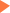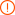2018SAT数学考点实例讲解 二元一次方程

2018年05月29日18:39 来源：小站教育作者：小站SAT编辑SAT数学二元一次方程考点实例

6(1-a)=3(a-b)+1 4(b-2)=3a, If(a,b)is the solution to the system above, then what is the value of a·b?

A.-1276/243 B.29/9 C.44/27 D.1276/243

6(1-a)=3(a-b)+1 → 6-6a=3a-3b+1 → -9a=-3b-5 → a=1/3·b+5/9

6(1-a)=3(a-b)+1 → 6-6a=3a-3b+1 → -9a=-3b-5 → a=1/3·b+5/9

then, substituting this result for a in the second equation gives

4(b-2)=3a → 4b-8=3(1/3·b+5/9) →4b-8=b+5/3 →3b=29/3·1/3→b=29/9

It then follows that:a=a=1/3(29/9)+5/9=44/27

The correct answer is : a·b=D.1276/243

2018SAT数学考点清单

专题推荐未输入验证码未输入验证码未输入验证码

SAT30天热搜沪公网安备 31010602002658号